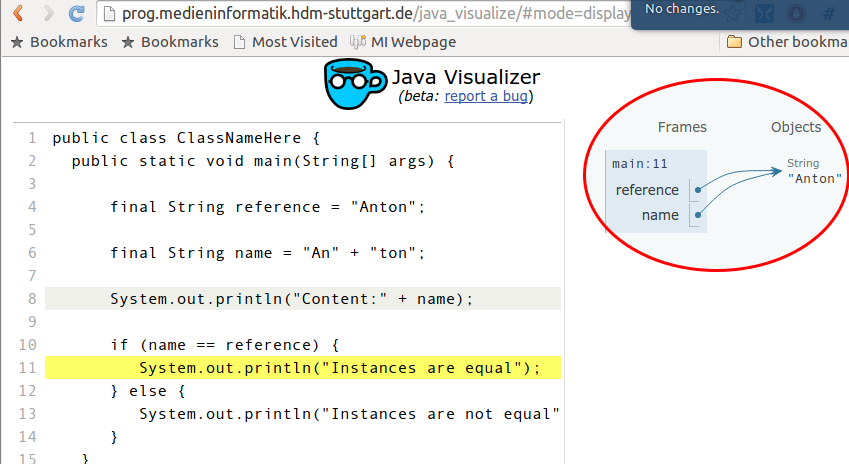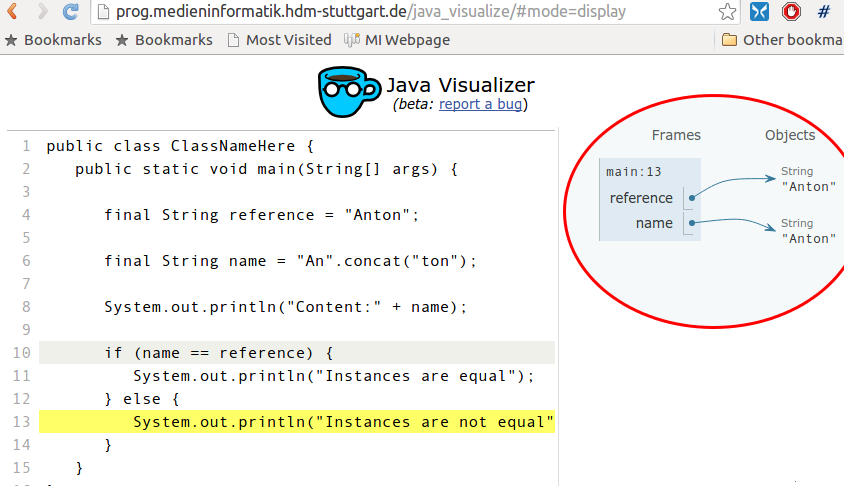## String exercisesNo. 135

### Strings on CodingBat

Q:

Solve all remaining exercises from the String-1 section and Warmup-1 > notString. Then solve the examples from the String-2 section.

### Tip

You may want to use your IDE and then copy/paste your code to the CodingBat web interface to avoid tedious syntax related fiddling.No. 136

 Q: Whenever you enter sensible information like billing details you don't want others sneaking these details. On the other hand you still want to recognize e.g. the account in question. One technique solving this conflict is masking. Considering an IBAN bank account number we have two possibilities: Full disclosure: IBAN: DE09300606010006778453 Advantage: Full control concerning e.g. correctness of entered value. Disadvantage: Possibility of tapping by criminals. Masking: IBAN: ##################8453 Advantage: Protection against felons. Disadvantage: Limited distinguishability. Implement a corresponding method:public class Mask { static private final int VISIBLE_POSITIONS = 4; /** *

Mask all but 4 positions of a given string by '#' characters. Examples:

* *
*
• "You" --> "You"
• *
• "Secret" --> "##cret"
• *
• "Ultimate test" --> "#########test"
• *
* * @param s The input string to be masked. * @return The masked string or null in case of null input string */ public static String mask(final String s) { ... return ... ; } }The following unit tests provide basic testing:public class MaskTest { /** * null input */ @Test public void testNullInput() { Assert.assertEquals( null, Mask.mask(null) ); } /** * 4 or less characters should be left untouched. */ @Test public void testBelowLimit() { Assert.assertEquals( "", Mask.mask("") ); Assert.assertEquals( "1", Mask.mask("1") ); Assert.assertEquals( "12", Mask.mask("12") ); Assert.assertEquals( "123", Mask.mask("123") ); Assert.assertEquals( "1234", Mask.mask("1234") ); } /** * More than 4 characters require masking. */ @Test public void testAboveLimit() { Assert.assertEquals( "#2345", Mask.mask("12345") ); Assert.assertEquals( "##3456", Mask.mask("123456") ); Assert.assertEquals( "###4567", Mask.mask("1234567") ); Assert.assertEquals( "####5678", Mask.mask("12345678") ); Assert.assertEquals( "#####6789", Mask.mask("123456789") ); } } A: Maven module source code available at P/Sd1/CoreClasses/StringMask. Notice the presence of an alternate implementation mask2(String s) employing yet not covered arrays being based on the Arrays.fill (...) library method.No. 137

### Analyzing strings

Q:

Consider the following string (excluding the comment):

73167176531330624919225119674426574742355349194934
96983520312774506326239578318016984801869478851843
85861560789112949495459501737958331952853208805511
12540698747158523863050715693290963295227443043557
66896648950445244523161731856403098711121722383113
62229893423380308135336276614282806444486645238749
30358907296290491560440772390713810515859307960866
70172427121883998797908792274921901699720888093776
65727333001053367881220235421809751254540594752243
52584907711670556013604839586446706324415722155397
53697817977846174064955149290862569321978468622482
83972241375657056057490261407972968652414535100474
82166370484403199890008895243450658541227588666881 ❶ (boldface highlighting)
16427171479924442928230863465674813919123162824586
17866458359124566529476545682848912883142607690042
24219022671055626321111109370544217506941658960408
07198403850962455444362981230987879927244284909188
84580156166097919133875499200524063689912560717606
05886116467109405077541002256983155200055935729725
71636269561882670428252483600823257530420752963450

The above sequence is meant to form a single string which has just been arranged in lines for convenience reasons.

In the above example the greatest product of four adjacent digits is 9 × 9 × 8 × 9 = 5832 due to the presence of 9989

Write a program that computes the maximum product value of 13 adjacent digits within the above string.

### Tip

1. Representing the above string in a Java program may be cumbersome due to its excessive length discouraging a single line representation. You may favor assembling it from smaller string literals:

final String input = "731671765 ... 94934"
+       ...
+        "716362695 ... 63450";
2. The String class does contain a method to retrieve a substring starting and ending at given index positions. Read its documentation to find it.

3. The String class does contain a method returning the char value at a given index. Read the documentation to find it.

4. Be careful with respect to overflow errors.

A:

We present a step by step solution. We start by coding a loop creating the set of all substrings of length 13. Reading the String documentation the method substring(...) allows for accessing the set of all substrings of 13 adjacent digits:

public static void main(String[] args) {
final String
input = "73167176531330624919225119674426574742355349194934"
+ "96983520312774506326239578318016984801869478851843"
+ "85861560789112949495459501737958331952853208805511"
+ "12540698747158523863050715693290963295227443043557"
+ "66896648950445244523161731856403098711121722383113"
+ "62229893423380308135336276614282806444486645238749"
+ "30358907296290491560440772390713810515859307960866"
+ "70172427121883998797908792274921901699720888093776"
+ "65727333001053367881220235421809751254540594752243"
+ "52584907711670556013604839586446706324415722155397"
+ "53697817977846174064955149290862569321978468622482"
+ "83972241375657056057490261407972968652414535100474"
+ "82166370484403199890008895243450658541227588666881"
+ "16427171479924442928230863465674813919123162824586"
+ "17866458359124566529476545682848912883142607690042"
+ "24219022671055626321111109370544217506941658960408"
+ "07198403850962455444362981230987879927244284909188"
+ "84580156166097919133875499200524063689912560717606"
+ "05886116467109405077541002256983155200055935729725"
+ "71636269561882670428252483600823257530420752963450";

final int NUM_OF_DIGITS = 13;   // The intended number of adjacent digits

for (int i = 0; i < input.length() - NUM_OF_DIGITS + 1; i++) {
final String thirteenDigitWord = input.substring(i, i + NUM_OF_DIGITS);
System.out.println(thirteenDigitWord);
}
}

This creates the following output:

7316717653133
3167176531330
1671765313306
...

5304207529634
3042075296345
0420752963450

The method charAt(int index) allows us to access each character within our string individually. We need to write a method which converts a digit like '4' to the value 4. Remember: The Unicode value of e.g. character '4' equals its ASCII value which is just 52. Thus we need a translation like:

private static int getDigitValue (final char digit) {
switch(digit) {
case '0': return 0;
case '1': return 1;
case '2': return 2;
case '3': return 3;
case '4': return 4;
case '5': return 5;
case '6': return 6;
case '7': return 7;
case '8': return 8;
case '9': return 9;

default:
System.err.println("Character '" + digit + "' is no digit, exiting");
System.exit(1);
return 0;
}
}

Since digit representations '0' to '9' appear »gapless« in strictly ascending order this may be simplified:

private static int getDigitValue (final char digit) {
return digit - '0';
}

Next we must compute a given string's product of digits. Taking the first value 7316717653133 from above we are looking for 7 × 3 ×1 × 6 ×7 × 1 ×7 × 6 × 5 × 3 × 1 × 3 × 3 = 5000940. We define:

private static int getDigitProduct(final String digitWord) {
int product = 1;
for (int i = 0; i < digitWord.length(); i++) {
product *= getDigitValue(digitWord.charAt(i));
}
return product;
}

Unfortunately this method sometimes returns weird results: The argument "5397536978179" for example returns -1594459696. This negative value is due to an arithmetic overflow: The product 5 × 3 × 9 × 7 × 5 × 3 × 6 × 9 × 7 × 8 × 1 × 7 × 9 is actually 2,700,507,600. This exceeds MAX_VALUE of 2,147,483,647 being representable by a 4 byte int variable.

Luckily a long variable will be able to hold positive values up to 9,223,372,036,854,775,807. This is much larger than our worst case 9 × 9 × 9 × 9 × 9 × 9 × 9 × 9 × 9 × 9 × 9 × 9 × 9 = 2,541,865,828,329 value. We thus have to change our data type from int to long:

private static long getDigitProduct(final String digitWord) {
long product = 1;
for (int i = 0; i < digitWord.length(); i++) {
product *= getDigitValue(digitWord.charAt(i));
}
return product;
}

Assembling these pieces together leaves us with the following main(...) method:

public static void main(String[] args) {

final String
input = "73167176531330624919225119674426574742355349194934"
+ "96983520312774506326239578318016984801869478851843"
+ "85861560789112949495459501737958331952853208805511"
+ "12540698747158523863050715693290963295227443043557"
+ "66896648950445244523161731856403098711121722383113" ❶
+ "62229893423380308135336276614282806444486645238749"
+ "30358907296290491560440772390713810515859307960866"
+ "70172427121883998797908792274921901699720888093776"
+ "65727333001053367881220235421809751254540594752243"
+ "52584907711670556013604839586446706324415722155397"
+ "53697817977846174064955149290862569321978468622482"
+ "83972241375657056057490261407972968652414535100474"
+ "82166370484403199890008895243450658541227588666881"
+ "16427171479924442928230863465674813919123162824586"
+ "17866458359124566529476545682848912883142607690042"
+ "24219022671055626321111109370544217506941658960408"
+ "07198403850962455444362981230987879927244284909188"
+ "84580156166097919133875499200524063689912560717606"
+ "05886116467109405077541002256983155200055935729725"
+ "71636269561882670428252483600823257530420752963450";

final int NUM_OF_DIGITS = 13;   // The intended number of adjacent digits

long maximumDigitProduct = 0;
String maxDigitString = null;
for (int i = 0; i < input.length() - NUM_OF_DIGITS + 1; i++) {
final String digitWord = input.substring(i, i + NUM_OF_DIGITS);
final long productOfDigits = getDigitProduct(digitWord);
if (maximumDigitProduct < productOfDigits) {
maximumDigitProduct = productOfDigits;
maxDigitString = digitWord;
}
}
System.out.println("The substring '" + maxDigitString +
"' yields the largest product value " + getDigitProduct(maxDigitString) + ".");
}

The largest product of 13 successive digits stems from the substring "5576689664895" ❶ starting with the last three digits in the fourth definition line of String input = ....

The substring '5576689664895' yields the largest product value 23514624000.No. 138

### Pitfalls using “==”: Equality of String instances

Q:

Consider the following code snippet:

public static void main(String[] args) {

final String a1 = "TestA", a2 = "TestA";
System.out.println("a1 == a2: " + (a1 == a2));
System.out.println("a1.equals(a2): " + a1.equals(a2));

final String b1 = new String("TestB"), b2 = new String("TestB");
System.out.println("b1 == b2: " + (b1 == b2));
System.out.println("b1.equals(b2): " + b1.equals(b2));
}

Execute this code and explain the resulting output.

### Tip

A:

Execution yields:

a1 == a2: true ❶
a1.equals(a2): true
b1 == b2: false ❷
b1.equals(b2): true
 The string literal "TestA" is being instantiated only once: The Java™ compiler implementation allocates only one instance of class String per string literal. The string literal "TestA" (even if being defined within different classes) always represents the same object. Thus the two distinct variables a1 and a2 are being assigned an identical reference pointing to this unique instance. As per definition the operator == compares two object references for equality and thus returns true. You might as well write: System.out.println("TestA" == "TestA");The method System.identityHashCode(Object o) returns Object.hashCode() rather then String.hashCode(). This hash code from java.lang.Object has a one to one correspondence to an object's reference and thus helps to understand the underlying object references: System.out.println("Hashcode a1 == " + System.identityHashCode(a1) + ", Hashcode a2 == " + System.identityHashCode(a2));Hashcode a1 == 366712642, Hashcode a2 == 366712642a1 and a2 thus likely contain an identical object reference. Using the equals() method for comparison indeed returns true. Every call to the String constructor will create a new string instance. Thus b1 and b2 will hold two distinct references pointing to different String instances albeit these two instances contain identical values. Following the above reasoning we may execute: System.out.println("Hashcode b1 == " + System.identityHashCode(b1) + ", Hashcode b2 == " + System.identityHashCode(b2));This yields different values corresponding to two different objects: Hashcode b1 == 1829164700, Hashcode b2 == 2018699554Comparing these two reference values for equality the “==” operator thus returns false. The equals() method however still returns a value of true since both distinct objects carry an identical value.No. 139

### Weird, weirder, weirdest!

Q:

Consider the following code snippet:

public static void main(String[] args) {

final String reference = "Anton";

final String name = "An" + "ton";

System.out.println("Content:" + name);

if (name == reference) {
System.out.println("Instances are equal");
} else {
System.out.println("Instances are not equal");
}
}

Execute the above code.

The following code differs only in the way the variable name ❶ is being defined:

public static void main(String[] args) {

final String reference = "Anton";

final String name = "An".concat("ton"); ❶

System.out.println("Content:" + name);

if (name == reference) {
System.out.println("Instances are equal");
} else {
System.out.println("Instances are not equal");
}
}

Explain the results.

### Tip

1. Think about compile time and run time considerations.

A:

Having name = "An" + "ton" in place we get:

Content:Anton
Instances are equal

The Java compiler performs a clever optimization: The expression "An" + "ton" is being evaluated at compile time. Its value is identical to the string literal "Anton" and thus both variables name and reference will be initialized to a common String instance:When starting from name = "An".concat("ton") the result is different:

Content:Anton
Instances are not equal

This time the Java compiler is unable to dynamically resolve the expression "An".concat("ton") at compile time. Instead the method concat(...)will be called at runtime resulting in a second String instance which by coincidence contains an identical value. Thus name and reference point to different objects: